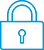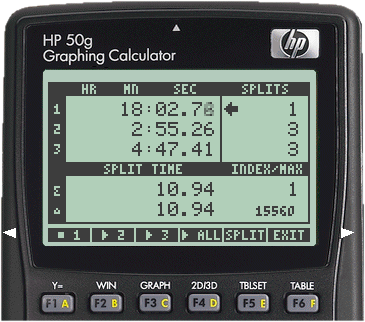cancel
Showing results for
Search instead for
Did you mean:It has been a while since anyone has replied. Simply ask a new question if you would like to start the discussion again.New member
2 0 0 0
Message 1 of 2
508
Flag Post

# Polynomial graphing and inequalitiesHP Recommended
HP 50g Graphing Calculator

Hello!

I have been trying to plot both the equation 0 = x^5-3x^3+2x^2-3 and the inequality x^5-3x^3+2x^2-3 > 0. However, the graph doesn't show in the screen regardless of how much I zoom out. I have checked flag 3 and tried to set up the plot to "Truth", but nothing works. What am I doing wrong?

Tags (1)
1 REPLY 1Level 6
140 139 29 60
Message 2 of 2
Flag PostHP Recommended

Not sure if this is your issue, but make sure that the plot parameter's independent variable (which defaults to an uppercase X) exactly matches what you've got in your equations.  That variable name is case-sensitive, so you need to make sure the equation's variable IDs are the same case as the plot parameter for the independent variable.  Your equations have all the x's as lowercase, so that could be part of the problem.

Hope this helps!

† The opinions expressed above are the personal opinions of the authors, not of HP. By using this site, you accept the Terms of Use and Rules of Participation